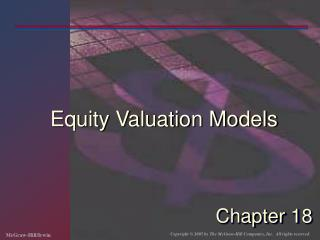DownloadDownload PresentationChapter 18

# Chapter 18

Télécharger la présentation## Chapter 18

- - - - - - - - - - - - - - - - - - - - - - - - - - - E N D - - - - - - - - - - - - - - - - - - - - - - - - - - -
##### Presentation Transcript

1. Equity Valuation Models Chapter 18

2. Models of Equity Valuation • Basic Types of Models • Balance Sheet Models • Dividend Discount Models • Price/Earning Ratios • Estimating Growth Rates and Opportunities

3. Intrinsic Value and Market Price • Intrinsic Value • Self assigned Value • Variety of models are used for estimation • Market Price • Consensus value of all potential traders • Trading Signal • IV > MP Buy • IV < MP Sell or Short Sell • IV = MP Hold or Fairly Priced

4. Dividend Discount Models: General Model V0 = Value of Stock Dt = Dividend k = required return

5. No Growth Model Stocks that have earnings and dividends that are expected to remain constant. Preferred Stock

6. No Growth Model: Example E1 = D1 = \$5.00 k = .15 V0 = \$5.00 / .15 = \$33.33

7. Constant Growth Model g = constant perpetual growth rate

8. Constant Growth Model: Example E1 = \$5.00 b = 40% k = 15% (1-b) = 60% D1 = \$3.00 g = 8% V0 = 3.00 / (.15 - .08) = \$42.86

9. Estimating Dividend Growth Rates g = growth rate in dividends ROE = Return on Equity for the firm b = plowback or retention percentage rate (1- dividend payout percentage rate)

10. Specified Holding Period Model PN = the expected sales price for the stock at time N N = the specified number of years the stock is expected to be held

11. Growth & No Growth Components of Value PVGO = Present Value of Growth Opportunities E1 = Earnings Per Share for period 1

12. Partitioning Value: Example ROE = 20% d = 60% b = 40% E1 = \$5.00 D1 = \$3.00 k = 15% g = .20 x .40 = .08 or 8%

13. Partitioning Value: Example Vo = value with growth NGVo = no growth component value PVGO = Present Value of Growth Opportunities

14. Price Earnings Ratios • P/E Ratios are a function of two factors • Required Rates of Return (k) • Expected growth in Dividends • Uses • Relative valuation • Extensive Use in industry

15. P/E Ratio: No Expected Growth • E1 - expected earnings for next year • E1 is equal to D1 under no growth • k - required rate of return

16. P/E Ratio with Constant Growth b = retention ratio ROE = Return on Equity

17. Numerical Example: No Growth E0 = \$2.50 g = 0 k = 12.5% P0 = D/k = \$2.50/.125 = \$20.00 PE = 1/k = 1/.125 = 8

18. Numerical Example with Growth b = 60% ROE = 15% (1-b) = 40% E1 = \$2.50 (1 + (.6)(.15)) = \$2.73 D1 = \$2.73 (1-.6) = \$1.09 k = 12.5% g = 9% P0 = 1.09/(.125-.09) = \$31.14 PE = 31.14/2.73 = 11.4 PE = (1 - .60) / (.125 - .09) = 11.4

19. Pitfalls in P/E Analysis • Use of accounting earnings • Historical costs • May not reflect economic earnings • Reported earnings fluctuate around the business cycle.

20. Other Valuation Ratios • Price-to-Book • Price-to-Cash Flow • Price-to-Sales

21. Inflation and Equity Valuation • Inflation has an impact on equity valuations. • Historical costs underestimate economic costs. • Empirical research shows that inflation has an adverse effect on equity values. • Research shows that real rates of return are lower with high rates of inflation.

22. Lower Equity Values with Inflation • Shocks cause expectation of lower earnings by market participants. • Returns are viewed as being riskier with higher rates of inflation. • Real dividends are lower because of taxes.